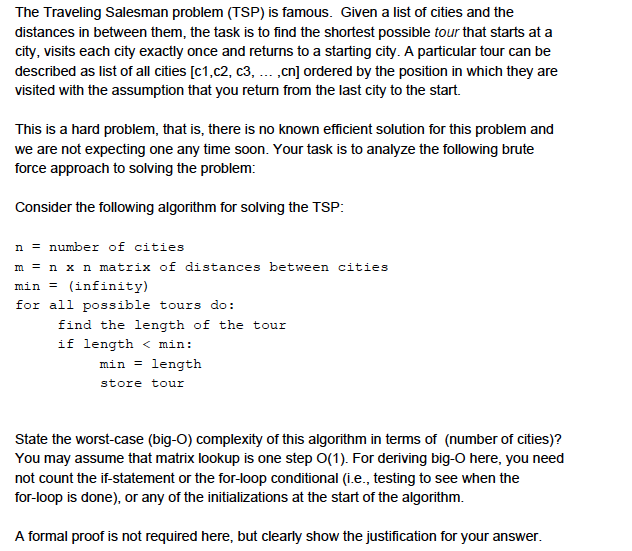# The Traveling Salesman problem (TSP) is famous. Given a list of cities and the distances in betwe...The Traveling Salesman problem (TSP) is famous. Given a list of cities and the distances in between them, the task is to find the shortest possible tour that starts at a city, visits each city exactly once and returns to a starting city. A particular tour can be described as list of all cities [c1,c2, c3, ,cn] ordered by the position in which they are visited with the assumption that you return from the last city to the start. This is a hard problem, that is, there is no known efficient solution for this problem and we are not expecting one any time soon. Your task is to analyze the following brute force approach to solving the problem: Consider the following algorithm for solving the TSP: n number of cities m-nx n matrix of distances between cities min(infinity) for all possible tours do: find the length of the tour if length

(a) Travelling Salesman Problem:

Given a set of cities and distance between every pair of cities, the problem is to find the shortest possible route or path that visits each city exactly once and returns to the starting point.

The problem is a famous NP hard problem in combinatorial optimization. Time complexity of travelling salesman is not polynomial but it is exponential. So, the problem is not solvable in polynomial time and hence, it comes under NP hard type problem.

Algorithm:

n=numbers of cities

m= n x n matrix of distances between cities

min=(infinity)

for all possible tours, do:

find the length of the tour

if length<min

min=length

store tour

Worst case (big O) time complexity of the given algorithm:

Solution to Travelling salesman problem:

Consider city 1 as the starting point and ending point.
Generate all (n-1)! Permutations of given cities.
Calculate cost of every permutation or tour and keep track of minimum cost permutation.
Return the permutation or tour with minimum cost.
Let the given set of cities be {1, 2, 3, 4,….n}. Let us consider city 1 as starting and ending point of output. For every other city i (other than 1), we find the minimum cost path with city 1 as the starting point, i as the ending point and all cities appearing exactly once.

Let the cost of this route be cost(i), the cost of corresponding cycle would be cost(i) + dist(i,1) where dist(i, 1) is the distance from city i to 1. Finally, we got the minimum of all [cost(i) + dist(i, 1)] values.

To calculate cost (i) of route using Dynamic Programming, we need to have some recursive relation in terms of sub-problems. Let us define a term C(S, i) be the cost of the minimum cost route visiting each city in set S exactly once, starting at 1 and ending at i.

We start with all subsets of size 2 and calculate the cost C(S, i) for all subsets where S is the subset, then we calculate cost C(S, i) for all subsets S of size 3 and so on. Note that city 1 must be present in every subset. Calculate by following method:

If size of S is 2, then S must be {1, i},

C(S, i) = dist(1, i)

Else if size of S is greater than 2.

C(S, i) = min { cost C(S-{i}, j) + dis(j, i)} where j belongs to S, j != i and j != 1.

For a set of size n, we consider n-2 subsets each of size n-1 such that all subsets don’t have nth in them. So, total no. of subproblems are:

##### Add Answer of: The Traveling Salesman problem (TSP) is famous. Given a list of cities and the distances in betwe...
Similar Homework Help Questions
• ### traveling salesman problem

In the classic traveling salesman problem, a salesman is expected to visit n cities, in each tour he is expected to visit each city only once. The question becomes, inwhich order should he visit the cities? The traveling salesman asks for the shortest route to visit a collection of cities. The aim is to minimize total distancetraveled? A common assumption used is that all tours and its reverse is symmetrical; that the distance AB is equal to BA where A...

• ### An Computer Programming lab consisting of using Graphs and C language

Need some one to help with the strategy and algorithms necessary. Basically any help at all would be useful including some bits of coding. If anyone has done this typeof programming before please help or give copy of code to study.Systems ProgrammingLab 2: C and GraphsOverviewThere are few better ways of getting acquainted with a language than to simply sit down and write some reasonably complex code, and so for this first lab we'll forgothe "systems" aspect of the course...

Need Online Homework Help?